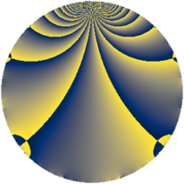# Properties

 Label 930.2.eLevel $930$ Weight $2$ Character orbit 930.e Rep. character $\chi_{930}(929,\cdot)$ Character field $\Q$ Dimension $64$ Newform subspaces $2$ Sturm bound $384$ Trace bound $2$

# Related objects

## Defining parameters

 Level: $$N$$ $$=$$ $$930 = 2 \cdot 3 \cdot 5 \cdot 31$$ Weight: $$k$$ $$=$$ $$2$$ Character orbit: $$[\chi]$$ $$=$$ 930.e (of order $$2$$ and degree $$1$$) Character conductor: $$\operatorname{cond}(\chi)$$ $$=$$ $$465$$ Character field: $$\Q$$ Newform subspaces: $$2$$ Sturm bound: $$384$$ Trace bound: $$2$$ Distinguishing $$T_p$$: $$47$$

## Dimensions

The following table gives the dimensions of various subspaces of $$M_{2}(930, [\chi])$$.

Total New Old
Modular forms 200 64 136
Cusp forms 184 64 120
Eisenstein series 16 0 16

## Trace form

 $$64q + 64q^{4} + 8q^{9} + O(q^{10})$$ $$64q + 64q^{4} + 8q^{9} - 4q^{10} + 64q^{16} + 16q^{19} + 20q^{25} - 24q^{31} + 8q^{36} - 8q^{39} - 4q^{40} - 32q^{45} - 72q^{49} - 8q^{51} + 64q^{64} + 16q^{66} - 16q^{69} - 32q^{70} + 16q^{76} + 48q^{81} - 52q^{90} + 8q^{94} + O(q^{100})$$

## Decomposition of $$S_{2}^{\mathrm{new}}(930, [\chi])$$ into newform subspaces

Label Dim. $$A$$ Field CM Traces $q$-expansion
$$a_2$$ $$a_3$$ $$a_5$$ $$a_7$$
930.2.e.a $$32$$ $$7.426$$ None $$-32$$ $$0$$ $$2$$ $$0$$
930.2.e.b $$32$$ $$7.426$$ None $$32$$ $$0$$ $$-2$$ $$0$$

## Decomposition of $$S_{2}^{\mathrm{old}}(930, [\chi])$$ into lower level spaces

$$S_{2}^{\mathrm{old}}(930, [\chi]) \cong$$ $$S_{2}^{\mathrm{new}}(465, [\chi])$$$$^{\oplus 2}$$